Function Repository Resource:

# L1Solve

Solve the linear least absolute value problem

Contributed by: Jan Mangaldan
 ResourceFunction["L1Solve"][m,b] finds an x that solves the linear least absolute value problem for the matrix equation m.x==b.

## Details

The linear least absolute value problem is also referred to as linear 1 norm minimization.
ResourceFunction["L1Solve"][m,b] gives a vector x that minimizes Norm[m.x-b,1].
All entries in the matrix m and the vector b must be real numbers.
SparseArray objects can be used in ResourceFunction["L1Solve"].
ResourceFunction["L1Solve"] takes the same options as LinearProgramming.

## Examples

### Basic Examples (1)

Solve a simple least absolute value problem:

 In:=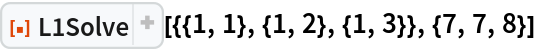Out=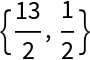### Scope (2)

Create a 4×3 matrix, and b is a length-4 vector:

 In:=Use exact arithmetic to find a vector x that minimizes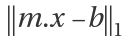:

 In:=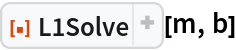Out=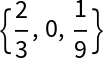Use machine arithmetic:

 In:=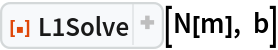Out=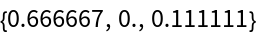Use 20-digit-precision arithmetic:

 In:=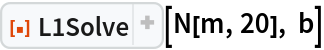Out=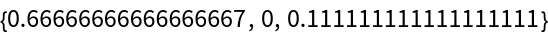Use a sparse matrix:

 In:=Out=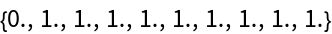### Applications (4)

Here is some data:

 In:=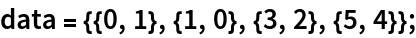Find the line that best fits the data in the least absolute deviation sense:

 In:=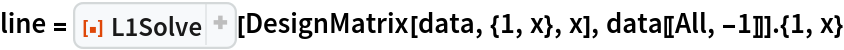Out=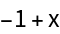Find the quadratic that best fits the data in the least absolute deviation sense:

 In:=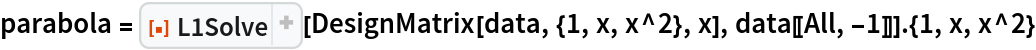Out=Show the data with the two curves:

 In:=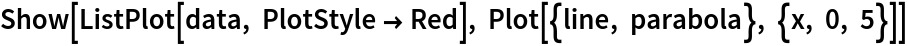Out=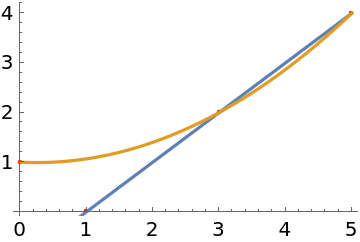### Properties and Relations (2)

For a vector b, L1Solve is equivalent to ArgMin[Norm[m.x-b,1],x]:

 In:=In:=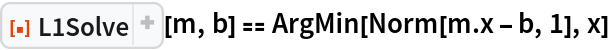Out=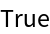Create a 5×2 matrix and a length-5 vector:

 In:=Solve the least absolute value problem:

 In:=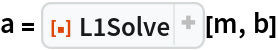Out=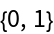This is the minimizer of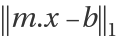:

 In:=Out=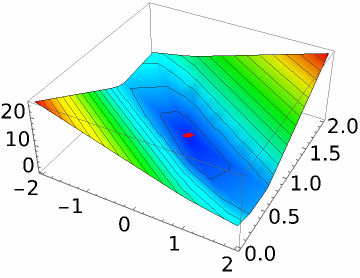It also gives the coefficients for the line with least absolute deviation from the points:

 In:=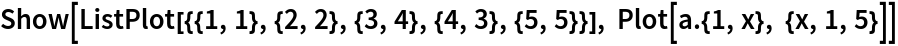Out=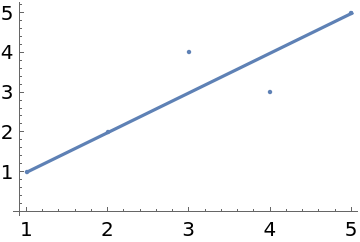## Version History

• 1.0.0 – 06 January 2021